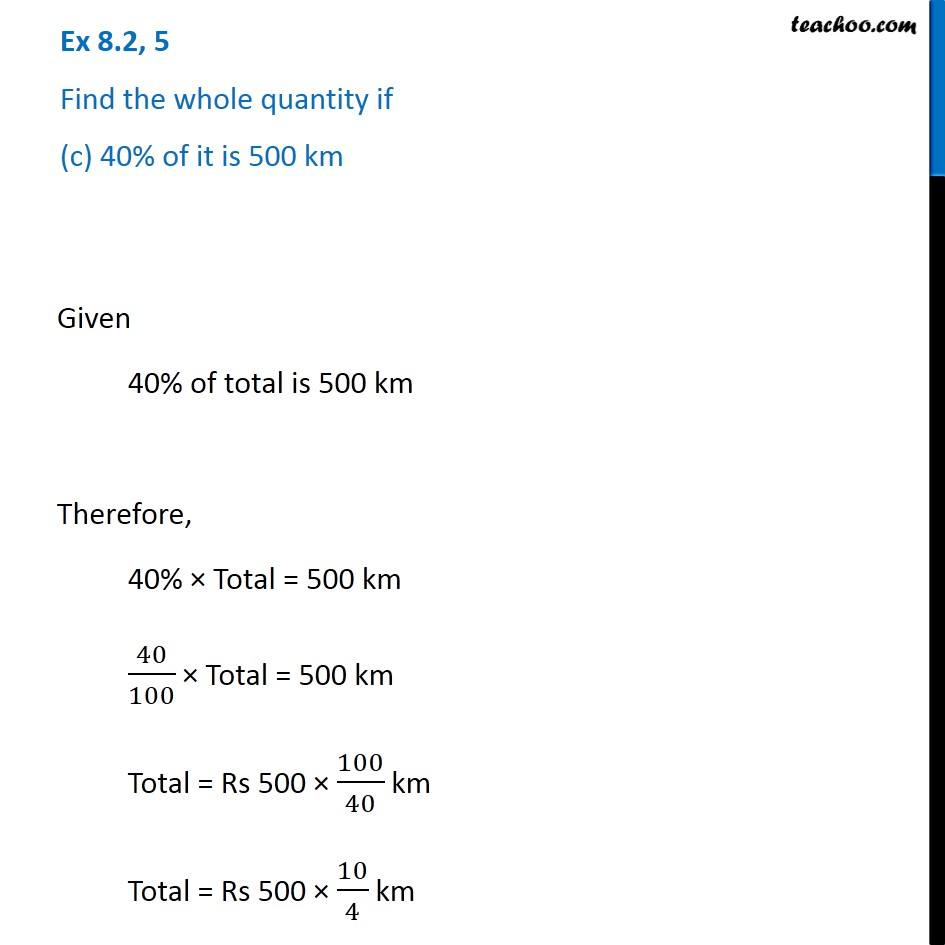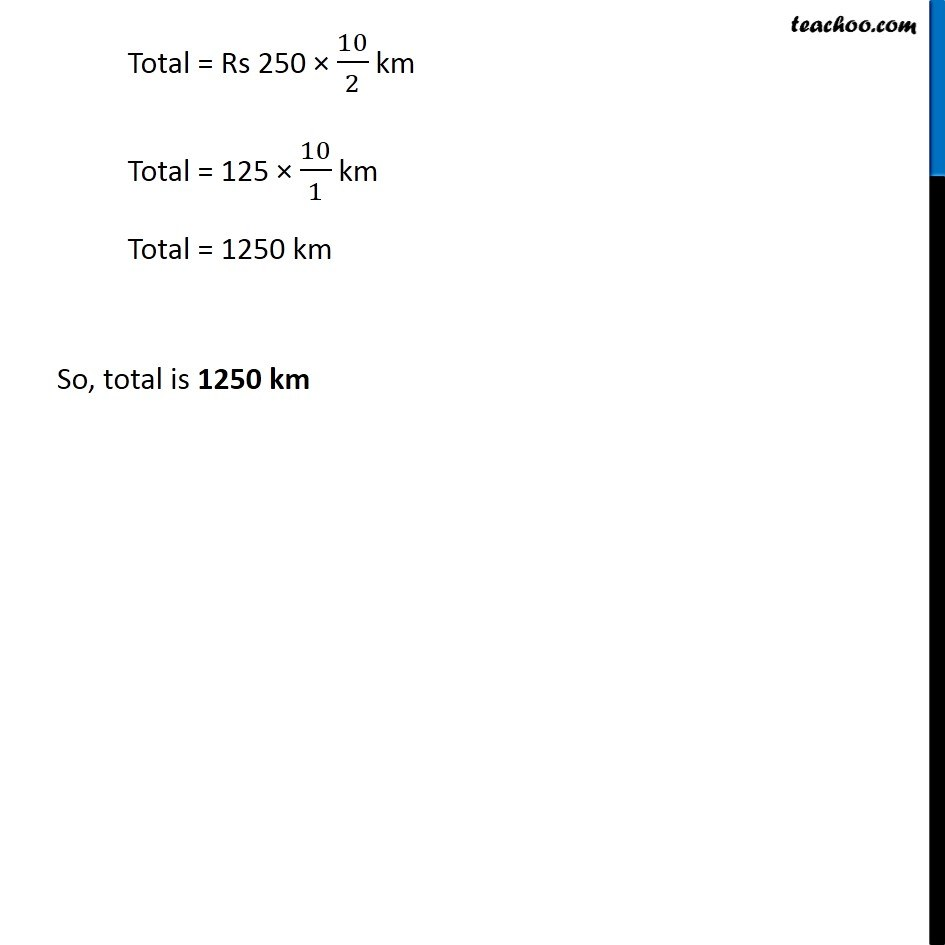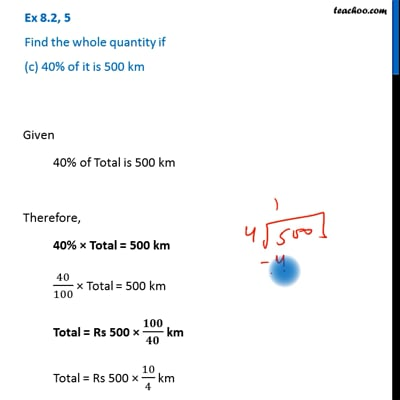Ex 8.2

Chapter 8 Class 7 Comparing Quantities
Serial order wiseThis video is only available for Teachoo black users

Get live Maths 1-on-1 Classs - Class 6 to 12

### Transcript

Ex 8.2, 5 Find the whole quantity if (c) 40% of it is 500 km Given 40% of total is 500 km Therefore, 40% × Total = 500 km 40/100 × Total = 500 km Total = Rs 500 × 100/40 km Total = Rs 500 × 10/4 km Total = Rs 250 × 10/2 km Total = 125 × 10/1 km Total = 1250 km So, total is 1250 km